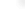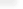Enhanced Typesetting Automation

Mathematica 7 introduces a new level of automation in mathematical typesetting. Building on two decades of experience, Mathematica 7 uses a large number of new algorithms and heuristics developed at Wolfram Research to generate traditional mathematical notation that exhibits the clarity and elegance of hand-crafted mathematics written by mathematical experts—but on an arbitrarily large scale.

Related Functions